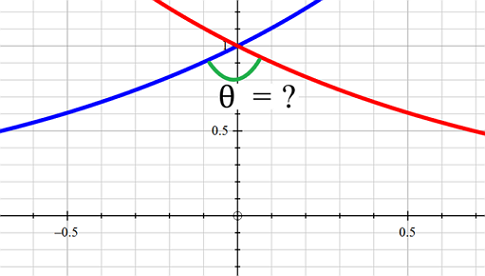# Does A Protractor Help?

Calculus Level 2What can we say about these two curves: $\large y_1=e^{x} \qquad \text{ and } \qquad y_2=e^{-x} \; ?$

×